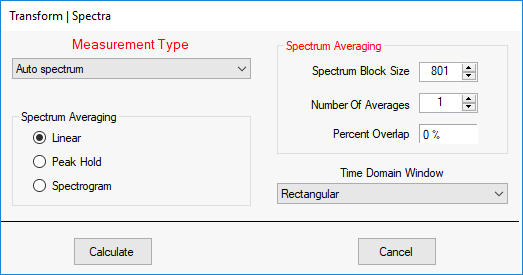# Transform | Spectra

Calculates Fourier spectra, Auto & Cross spectra, PSD's or ESD's from time waveforms or frequency spectra.  When this command is executed, the dialog box below is opened.All of the Data Blocks open in the Work Area are listed in this window.  Only certain data can be used for calculating each type of spectrum.

• A Data block with Time waveforms is required to calculate Fourier Spectra, Cross Spectra, or a Spectrogram

• A Data Block with single channel (Input, Output, or Both) M#s is required to calculate Auto Spectra, PSD's, or ESD's.

## Fourier spectrum

• A Fourier spectrum is merely the DFT of a time waveform

• The FFT calculates the Digital Fourier Spectrum (DFT ) of each M# in a Data Block.

• See The FFT and DFT section for details.

## Auto spectrum

• An Auto spectrum is calculated by averaging together multiple Auto spectrum estimates.

• Each Auto spectrum estimate is calculated by multiplying a DFT by its complex conjugate.

• The DFT is complex valued, but the Auto spectrum is only Real valued (magnitude only).

## Cross spectrum

• A Cross spectrum is calculated by averaging together multiple Cross spectrum estimates.

• Each Cross spectrum estimate is calculated by multiplying the DFT of an Output M# by the complex conjugate of the DFT of a different Input M#.

• The Cross spectrum is complex valued.

## Power Spectral Density (PSD)

• A PSD is an Auto spectrum that has been "normalized" by dividing it by the frequency resolution of the Auto spectrum.

• If the units of an Auto spectrum are g^2, the units of its corresponding PSD are g^2 / Hz.

## Energy Spectral Density (ESD)

• ESD's are used to characterize transient signals.

• An ESD is a PSD multiplied by the time length (T) of the time domain signal used to create the ESD.

• If the units of a PSD are g^2 / Hz, the units of its corresponding ESD are (g^2 - sec) / Hz.

## Time Waveform File

If you choose a Time Waveform File (TWF), time domain windowing, overlap processing, and spectrum averaging can be used to calculate spectral estimates.

## Spectrogram

• A Spectrogram is a series of spectra that are calculated from short time portions of a long time waveform

• If the Number of Averages = 10 during Spectrum Averaging, 10 individual spectrum estimates are retained as a Spectrogram instead of averaging the 10 spectral estimates together.